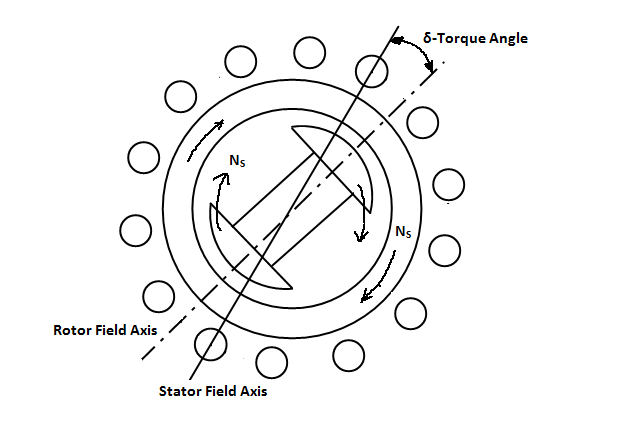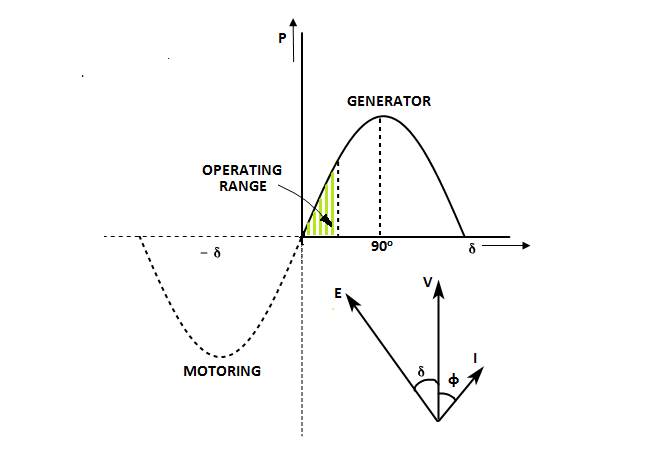# Torque Angle versus Load or Power Angle:

These three terms Torque angle, Power angle and Load angle are associated with Synchronous machines that are synchronous generator and synchronous motor. They named as Synchronous machines as they revolve at synchronous speed. First of all we will see what the synchronous speed is.

Synchronous Speed:

For a given number of poles and frequency of a system the Synchronous speed is constant and is given by

Ns=120 f/p

Where

Ns= Synchronous speed in RPM

f= frequency

p= No. of Poles of Alternator

### Now we will see what the Torque angle is?

Torque angle is generally defined for Synchronous motor.

## Torque angle for Synchronous Motor:

Torque angle δ is the angle between Rotor flux and Stator fluxes, both are rotating at synchronous speed. It is noted that for synchronous motors the rotor flux axis lags the stator flux axis by the angle δ as shown in figure.Synchronous Motor with Stator and Rotor Fluxes

The stator of the synchronous motor consists of three phase armature windings. When these windings supplied with three-phase supply, a constant magnetic field which revolves at synchronous speed Ns. is produced. Synchronous motor rotor produces a constant flux with the help of DC Excitation.

These two fluxes interact with each other and a torque is developed called the electromagnetic torque given by

Te proportional to (stator field strength) * (rotor field strength) * Sin δ

Where δ is the torque angle between stator and rotor magnetic poles.

If the angle δ is expressed as function of time then δ=ωt

Now the torque expression becomes

Te proportional to (stator field strength) * (rotor field strength) * Sin ωt

The torque varies sinusoidally with time. Its value reverses during each half cycle; average value of this torque over a complete cycle is zero. Thus, a synchronous motor has no self starting torque.

To make the motor starting the unexcited rotor is speeded up to synchronous speed by some arrangement and then excited by DC source. The moment this synchronously rotating rotor is excited, it is magnetically locked into position with the stator i.e., the rotor poles are now engaged with the stator poles and both run synchronously in the same direction.

As the load on the motor is increased, the torque angle also increases and motor still running at synchronous speed. The value of the torque angle depends on the amount of load to be met by the motor. In other words, the torque developed by the motor depends on torque angle.

### Now we will see what the Power or Load angle is?

Well, the power or load angle is defined for Synchronous Machine and also for Transmission Lines.

When the synchronous machine is connected to an infinite bus, its speed and terminal voltage are fixed and unalterable. The control variables are only field current and the mechanical torque on the shaft.

The figure below represents the variation of power P with Power angle δ. This power versus load angle curve has a sinusoidal shape and is usually called the power-angle characteristic curve of the synchronous machine. The power P, for generator is taken as positive and therefore, for motor as negative.Power-Angle Curve of Synchronous Machine

## Power or Load angle for Generator:

The power angle is defined as the angle between Induced EMF and Terminal voltages.

For Generator action E leads V.

Where

P = power generated per phase, watts.

|E| = Induced EMF per phase, volts.

|V|= Terminal voltage per phase, volts

δ = Power angle, Angle between E and V vectors (Considered Positive for Generator)

X = Synchronous reactance of Generator, Ohms

The typical Load angle is around 30o Electrical. With increased MW load the load angle also increases and the generator delivers more power.

The only way to vary load angle is by varying the input to the Turbine. The power output of a synchronous Generator can be changed by changing its mechanical power input. In case of alternators to deliver desired output for variable loads, Governor is used. Speed control governor maintain the turbine speed constant by varying the input (Steam or Gas or Water depends on type of Prime mover or Turbine) to the Prime mover. Governors take feedback of load on the generator and according to feed input to the turbine.

Change in excitation using Static Excitation or Brushless Excitation System gives only the change in EMF and Reactive power supplied by the machine.

Load angle or Power angle for Motoring Action:

The power angle is defined as the angle between Induced Back EMF and applied stator voltages.

For motor action E lags V.

Where

P = Mechanical equivalent power produced per phase, watts.

|E| = Induced Back EMF per phase, volts.

|V|= Supply voltage per phase, volts

δ = Power angle, Angle between E and V vectors (Considered Negative for Motor)

X = Synchronous reactance of Motor, Ohms

### What are the limits of Power angle?

The system is stable only if the power angle δ is between -90° and +90° where the slope dP/ dδ is positive, that is the range in which an increase in power angle results in an increase in transmitted power.

Beyond this range generator or motor comes out of synchronism and results loss of stability. Loss of stability leads to stalling of synchronous motor when that motor is supplied with synchronous generator. High fluctuation of current and voltage within the transmission network when two synchronous generators are connected. The power transfer between the sources is alternatively positive and negative with an average of zero.

Loss of synchronism is called loss of stability. If a synchronous machine losses synchronism, it is tripped automatically by the loss of synchronism protection and is not allowed to rotate asynchronously.

## Power or Load angle for Transmission Line:

The load angle is defined as the angle between sending Vs and receiving Vr end voltages.

The power transfer of AC transmission lines is related with the rated voltage as follows.

Where

P = Power transfer per phase, watts

|VS|= Sending end voltage, Volts per phase.

|VR|= Receiving end voltage, Volts per phase.

δ = Power angle, Angle between VS and VR vectors.

X = Reactance of transmission Line, Ohms

### Conclusion:

Although these three terms are using as synonymous to each other by many authors, the Torque angle is used to assess the performance of individual synchronous machine. Whereas, if one or more synchronous machines connected to infinite bus bars then the term Power angle is used.

## 1 thought on “Torque Angle versus Load or Power Angle:”

1.Richard kapolyo

Good explanation